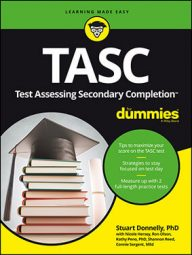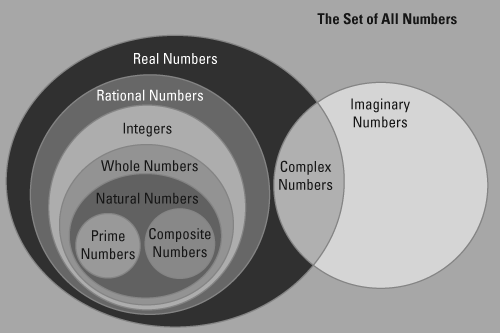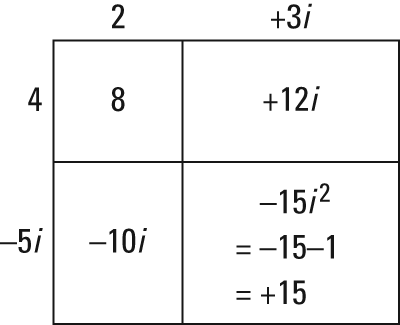##### TASC For DummiesWhile most questions on the TASC Math exam require you to deal with real numbers, you'll probably run into one or two problems that involve complex numbers.

The first time most people encounter complex numbers is in algebra, when they find out that it's possible to take the square root of negative numbers. The important thing to remember here is that

This means, for example, that

Complex numbers aren't just numbers that occur when taking the square root of negative numbers, though. They include any number that can be represented in the form a + bi, where a is the real part and bi is the imaginary part. This means any real number is a complex number when b = 0.

Using this definition, the Venn diagram shown here illustrates how complex numbers are the intersection of real numbers and imaginary numbers.Venn diagram of the classifications of numbers that includes complex and imaginary numbers.

Because complex numbers are still numbers, you can perform arithmetic operations with them, such as adding, subtracting, multiplying, and dividing.

When you add or subtract two complex numbers, you combine (add or subtract) the real parts together and the complex parts together.

Example: (4 + 2i) + (5 + 8i) = (4 + 5) + (2 + 8)i = 9 + 10i

Example: (9 + 5i) – (11 – 2i) = (9 – 11) + (5 – –2)i = –2 + 7i

When multiplying two complex numbers, treat them more like polynomials than traditional numbers. This means you have to do double distribution. The box method is useful here because it keeps you organized and helps prevent losing terms. To perform multiplication using the box method, separate out each term of the complex number either along the side or on top of the box. To fill in each inside the box, multiply the column header by the row header. Lastly, you need to combine like terms (the two terms that have i in them).

Take a look at this example: (2 + 3i)(4 – 5i)The box method can be used to make multiplying complex numbers easy.

Thus (2 + 3i)(4 – 5i) = 8 + 12i – 10i + 15 = 23 + 2i

Dividing two complex numbers would look like this:

To perform this division problem, you multiply both the top and the bottom of the quotient by the complex conjugate of the denominator. The complex conjugate of the denominator looks like the original denominator but with the opposite sign, so you would multiply the original question by:

This results in a rational denominator.

Try working through this example:

Multiply as if they are regular fractions:

Now multiply these two complex numbers:

Simplify and you get this solution:

This tells you that the real number part of the answer is

and the imaginary part is

Stuart Donnelly, PhD, was awarded a PhD in mathematics from Oxford University. He has prepared students for the TASC test and GED Test for the past two decades.

Stuart Donnelly, PhD, earned his doctorate in mathe-matics from Oxford University at the age of 25. Since then, he has established successful tutoring services in both Hong Kong and the United States and is considered by leading educators to be one of the most experienced and qualified private tutors in the country.

Stuart Donnelly, PhD, was awarded a PhD in mathematics from Oxford University. He has prepared students for the TASC test and GED Test for the past two decades.

Stuart Donnelly, PhD, was awarded a PhD in mathematics from Oxford University. He has prepared students for the TASC test and GED Test for the past two decades.

Sandra Luna McCune, PhD, is professor emeritus and a former Regents professor at Stephen F. Austin State University. She's now a full-time author. Shannon Reed, MA, MFA, is a visiting lecturer at the University of Pittsburgh, where she teaches composition, creative writing, and business writing.

Stuart Donnelly, PhD, was awarded a PhD in mathematics from Oxford University. He has prepared students for the TASC test and GED Test for the past two decades.